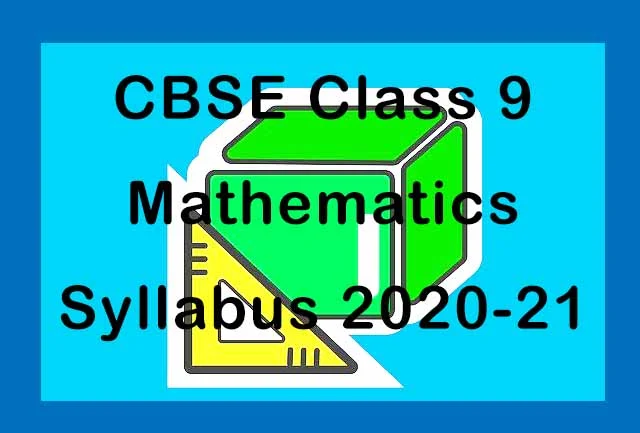## CBSE Class 9 Maths Syllabus 2020-21| Check Latest Exam Pattern

CBSE has released revised Class 9 Maths Syllabus 2020-21. The board has decided to reduce the syllabus due to coronavirus pandemic. There are various topics which have been deleted from the syllabus from academic session 2020-21. The question paper will be of 80 marks while 20 marks will be given on the basis of Internal Assessment which includes Pen Paper Test and Multiple Assessment, Portfolio and Lab Practical.Units Unit Name Marks I Number Systems 08 II Algebra 17 III Coordinate Geometry 04 IV Geometry 28 V Mensuration 13 VI Probability 10 - Total 80
Unit - I - Number Systems

• Real Numbers

Unit - II - Algebra

• Polynomials
• Linear Equations in two variables

Unit - III - Coordinate Geometry

• Coordinate Geometry

Unit - IV - Geometry

• Lines and Angles
• Triangles
• Circles
• Constructions

Unit - V - Mensuration

• Areas
• Surfaces, Areas and Volumes

Unit - VI - Statistics & Probability

• Statistics
• Probability

Topics that have been removed from Class 9 Maths Syllabus 2020-21

• Real Numbers - → Representation of terminating / non-terminating recurring decimals on the number line through successive magnification.
→ Explaining that every real number is represented by a unique point on the number line and conversely, viz. every point on the number line represents a unique real number.
→ Definition of nth root of a real number.

• Polynomials - → Motivate and State the Remainder Theorem with examples. Statement and proof of the Factor Theorem.
→ x3+y3+z3 -3xyz

• Pair of linear equations in two variables - Examples, problems on Ratio and Proportion.

• Coordinate geometry - No Deletion

• Introduction to Euclid's Geometry - Chapter Deleted

• Lines and Angles - No Deletion

• Triangles - → Proof of the theorem deleted- Two triangles are congruent if any two angles and the included side of one triangle is equal to any two angles and the included side of the other triangle (ASA Congruence).
→ Topic Deleted-Triangle inequalities and relation between ‘angle and facing side' inequalities in triangles.

• Area - Chapter Deleted

• Circles - → There is one and only one circle passing through three given non-collinear points.
→ If a line segment joining two points subtends equal angle at two other points lying on the same side of the line containing the segment, the four points lie on a circle.

• Constructions - → Construction of a triangle of given perimeter and base angles.

• Areas - → Application of Heron’s Formula in finding the area of a quadrilateral.

• Surfaces, Areas and Volumes - No Deletion

• Statistics - → Histograms (with varying base lengths),
→ Frequency polygons.
→ Mean, median and mode of ungrouped data.

• Probability - No Deletion

Prescribed Books:

• NCERT Maths Textbook - Class IX
• Laboratory Manual - Mathematics, secondary stage - NCERT Publication
• Mathematics Exemplar problems for class IX, NCERT publication